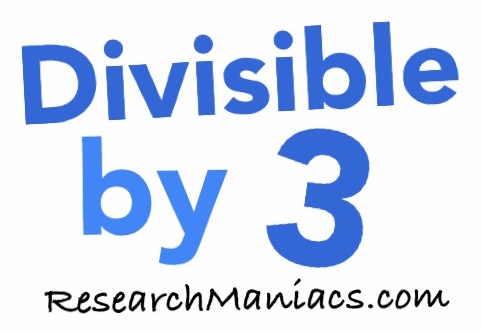Is 30 divisible by 3?Is 30 divisible by 3? In other words, if you divide 30 by 3, will you get a whole number with no remainder?

Of course, you could use a calculator to find out if 30 is divisible by 3, but what fun would that be?

To find out if 30 is divisible by 3, we will add up the numbers that make 30 as follows: 3 + 0 = 3

We know that if the sum of the numbers that make up 30 is divisible by 3, then 30 is divisible by 3.

Since the sum of the digits in 30 is divisible by 3, 30 is also divisible by 3. Thus, the answer to the question "Is 30 divisible by 3?" is as follows:

Yes

Note: If you divide 30 by 3, you get 10 which is a whole number.

Divisible by 3?
Do you need to check another number? Enter a number below to see if it is divisible by 3.

Is divisible by 3?

Is 31 divisible by 3?
Can you do it on your own now? If not, go here for the next number on our list.